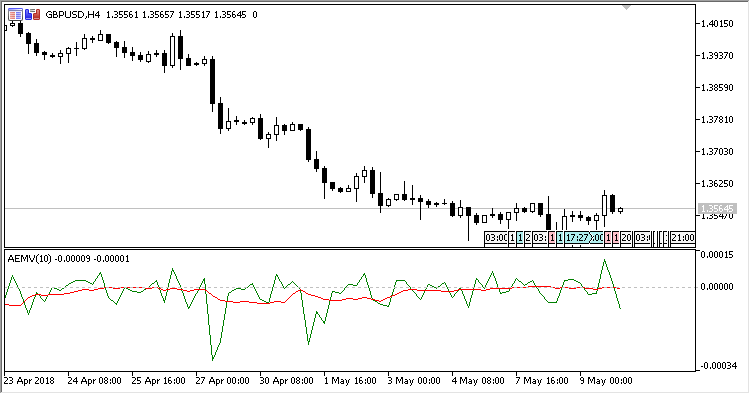# EMV – indicator for MetaTrader 5

• A+
category：MT5 INDICATORS

Indicator Arms' Ease of Movement Value (EMV) developed by Richard W. Arms Junior. It considers the volume and size of changing the price to evaluate its moving quantitatively for ease or complexity.

It has two input parameters:

• Period - calculation period;
• Method - calculation method.

Calculations:

`EMV = (((Current High + Current Low)/2) - ((Previous High + Previous Low)/2)) / (Current Volume/(Current High - Current Low))`